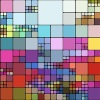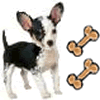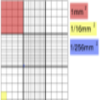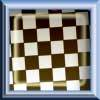# Search by Topic

#### Resources tagged with Interactivities similar to Napoleon's Theorem:

Filter by: Content type:
Age range:
Challenge level:

### There are 109 results

Broad Topics > Information and Communications Technology > Interactivities### Napoleon's Theorem

##### Age 14 to 18 Challenge Level:

Triangle ABC has equilateral triangles drawn on its edges. Points P, Q and R are the centres of the equilateral triangles. What can you prove about the triangle PQR?### Squaring the Rectangle

##### Age 14 to 18 Challenge Level:

Can you find a way to turn a rectangle into a square?### Tessellation Interactivity

##### Age 7 to 16 Challenge Level:

An environment that enables you to investigate tessellations of regular polygons### Excel Interactive Resource: Fraction Multiplication

##### Age 11 to 16 Challenge Level:

Use Excel to explore multiplication of fractions.### Excel Interactive Resource: Multiples Chain

##### Age 11 to 16 Challenge Level:

Use an interactive Excel spreadsheet to investigate factors and multiples.### Ratio Pairs 3

##### Age 11 to 16 Challenge Level:

Match pairs of cards so that they have equivalent ratios.### Loci Resources

##### Age 14 to 16 Challenge Level:

This set of resources for teachers offers interactive environments to support work on loci at Key Stage 4.### Excel Interactive Resource: Interactive Division

##### Age 11 to 16 Challenge Level:

Use an Excel to investigate division. Explore the relationships between the process elements using an interactive spreadsheet.### Factors and Multiples - Secondary Resources

##### Age 11 to 16 Challenge Level:

A collection of resources to support work on Factors and Multiples at Secondary level.### Percentages for Key Stage 4

##### Age 14 to 16 Challenge Level:

A group of interactive resources to support work on percentages Key Stage 4.### Excel Interactive Resource: Number Grid Functions

##### Age 11 to 16 Challenge Level:

Use Excel to investigate the effect of translations around a number grid.### Excel Interactive Resource: the up and Down Game

##### Age 11 to 16 Challenge Level:

Use an interactive Excel spreadsheet to explore number in this exciting game!### Excel Interactive Resource: Long Multiplication

##### Age 11 to 16 Challenge Level:

Use an Excel spreadsheet to explore long multiplication.### Countdown Fractions

##### Age 11 to 16 Challenge Level:

Here is a chance to play a fractions version of the classic Countdown Game.### Excel Interactive Resource: Equivalent Fraction Bars

##### Age 11 to 16 Challenge Level:

A simple file for the Interactive whiteboard or PC screen, demonstrating equivalent fractions.### Cushion Ball Interactivity

##### Age 14 to 16 Challenge Level:

The interactive diagram has two labelled points, A and B. It is designed to be used with the problem "Cushion Ball"### Excel Interactive Resource: Haitch

##### Age 11 to 16 Challenge Level:

An Excel spreadsheet with an investigation.### Spinners Environment

##### Age 5 to 18 Challenge Level:

A tool for generating random integers.### Solving with Euclid's Algorithm

##### Age 14 to 18 Challenge Level:

A java applet that takes you through the steps needed to solve a Diophantine equation of the form Px+Qy=1 using Euclid's algorithm.### White Box

##### Age 7 to 18 Challenge Level:

This game challenges you to locate hidden triangles in The White Box by firing rays and observing where the rays exit the Box.### Red or Black Spinner

##### Age 3 to 16 Challenge Level:

A simple spinner that is equally likely to land on Red or Black. Useful if tossing a coin, dropping it, and rummaging about on the floor have lost their appeal. Needs a modern browser; if IE then at. . . .### Excel Interactive Resource: Fraction Addition & Fraction Subtraction

##### Age 11 to 16 Challenge Level:

Use Excel to practise adding and subtracting fractions.### Attractive Tablecloths

##### Age 14 to 16 Challenge Level:

Charlie likes tablecloths that use as many colours as possible, but insists that his tablecloths have some symmetry. Can you work out how many colours he needs for different tablecloth designs?### Colour in the Square

##### Age 7 to 16 Challenge Level:

Can you put the 25 coloured tiles into the 5 x 5 square so that no column, no row and no diagonal line have tiles of the same colour in them?### Square Coordinates

##### Age 11 to 14 Challenge Level:

A tilted square is a square with no horizontal sides. Can you devise a general instruction for the construction of a square when you are given just one of its sides?### Inside Out

##### Age 14 to 16 Challenge Level:

There are 27 small cubes in a 3 x 3 x 3 cube, 54 faces being visible at any one time. Is it possible to reorganise these cubes so that by dipping the large cube into a pot of paint three times you. . . .### Overlap

##### Age 11 to 14 Challenge Level:

A red square and a blue square overlap so that the corner of the red square rests on the centre of the blue square. Show that, whatever the orientation of the red square, it covers a quarter of the. . . .### Using the Haemocytometer

##### Age 14 to 16 Challenge Level:

Practise your skills of proportional reasoning with this interactive haemocytometer.### Rollin' Rollin' Rollin'

##### Age 11 to 14 Challenge Level:

Two circles of equal radius touch at P. One circle is fixed whilst the other moves, rolling without slipping, all the way round. How many times does the moving coin revolve before returning to P?### Semi-regular Tessellations

##### Age 11 to 16 Challenge Level:

Semi-regular tessellations combine two or more different regular polygons to fill the plane. Can you find all the semi-regular tessellations?### Fractions and Percentages Card Game

##### Age 11 to 16 Challenge Level:

Match the cards of the same value.### Olympic Magic

##### Age 14 to 16 Challenge Level:

in how many ways can you place the numbers 1, 2, 3 … 9 in the nine regions of the Olympic Emblem (5 overlapping circles) so that the amount in each ring is the same?### Polycircles

##### Age 14 to 16 Challenge Level:

Show that for any triangle it is always possible to construct 3 touching circles with centres at the vertices. Is it possible to construct touching circles centred at the vertices of any polygon?### Diagonal Dodge

##### Age 7 to 14 Challenge Level:

A game for 2 players. Can be played online. One player has 1 red counter, the other has 4 blue. The red counter needs to reach the other side, and the blue needs to trap the red.### Squaring the Circle and Circling the Square

##### Age 14 to 16 Challenge Level:

If you continue the pattern, can you predict what each of the following areas will be? Try to explain your prediction.### A Tilted Square

##### Age 14 to 16 Challenge Level:

The opposite vertices of a square have coordinates (a,b) and (c,d). What are the coordinates of the other vertices?### Square It

##### Age 11 to 16 Challenge Level:

Players take it in turns to choose a dot on the grid. The winner is the first to have four dots that can be joined to form a square.### Disappearing Square

##### Age 11 to 14 Challenge Level:

Do you know how to find the area of a triangle? You can count the squares. What happens if we turn the triangle on end? Press the button and see. Try counting the number of units in the triangle now. . . .### Bow Tie

##### Age 11 to 14 Challenge Level:

Show how this pentagonal tile can be used to tile the plane and describe the transformations which map this pentagon to its images in the tiling.### Khun Phaen Escapes to Freedom

##### Age 11 to 14 Challenge Level:

Slide the pieces to move Khun Phaen past all the guards into the position on the right from which he can escape to freedom.##### Age 14 to 16 Challenge Level:

A counter is placed in the bottom right hand corner of a grid. You toss a coin and move the star according to the following rules: ... What is the probability that you end up in the top left-hand. . . .### Jam

##### Age 14 to 16 Challenge Level:

To avoid losing think of another very well known game where the patterns of play are similar.### Icosian Game

##### Age 11 to 14 Challenge Level:

This problem is about investigating whether it is possible to start at one vertex of a platonic solid and visit every other vertex once only returning to the vertex you started at.### Proof Sorter - Quadratic Equation

##### Age 14 to 18 Challenge Level:

This is an interactivity in which you have to sort the steps in the completion of the square into the correct order to prove the formula for the solutions of quadratic equations.### Sliding Puzzle

##### Age 11 to 16 Challenge Level:

The aim of the game is to slide the green square from the top right hand corner to the bottom left hand corner in the least number of moves.### Rolling Around

##### Age 11 to 14 Challenge Level:

A circle rolls around the outside edge of a square so that its circumference always touches the edge of the square. Can you describe the locus of the centre of the circle?### Guesswork

##### Age 14 to 16 Challenge Level:

Ask a friend to choose a number between 1 and 63. By identifying which of the six cards contains the number they are thinking of it is easy to tell them what the number is.### Konigsberg Plus

##### Age 11 to 14 Challenge Level:

Euler discussed whether or not it was possible to stroll around Koenigsberg crossing each of its seven bridges exactly once. Experiment with different numbers of islands and bridges.### Partitioning Revisited

##### Age 11 to 14 Challenge Level:

We can show that (x + 1)² = x² + 2x + 1 by considering the area of an (x + 1) by (x + 1) square. Show in a similar way that (x + 2)² = x² + 4x + 4### Tilted Squares

##### Age 11 to 14 Challenge Level:

It's easy to work out the areas of most squares that we meet, but what if they were tilted?